Understanding Julia and Mandelbrot Sets Karl Sims Julia set fractals are normally generated by initializing a complex number  z = x + yi  where  i2 = -1  and x and y are image pixel coordinates in the range of about -2 to 2. Then, z is repeatedly updated using:  z = z2 + c  where c is another complex number that gives a specific Julia set. After numerous iterations, if the magnitude of z is less than 2 we say that pixel is in the Julia set and color it accordingly. Performing this calculation for a whole grid of pixels gives a fractal image.Folding a Circle into a Julia Set

This process can be better understood visually by repeatedly transforming a shape using the inverse equation z = sqrt(z - c). The square root of a complex number halves its angle and square-roots its magnitude. Halving the angle of a shape is like collapsing a full circle into a half circle, as if a 360° fan is folded up halfway into a 180° fan. The other half is filled in the same way to make a duplicate, so it's more like folding a 720° fan with two overlapping copies of the shape into a 360° fan. (Mathematically, these two halves are the plus and minus results of the square root function.) When we square-root the magnitude it causes contraction towards the radius of 1, and expansion at the origin. Here is one iteration of this transformation applied step by step to a circle of radius 2 with a polar grid drawn on it. For this example c = .274 - .008i.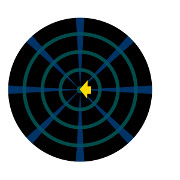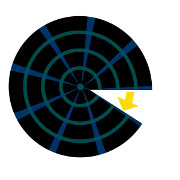Start with a circle Shift by -c Fold into half circle Same for theother half Square-rootthe magnitude

When this transformation is applied over and over again, a fractal shape emerges. Here is the next iteration starting from the result above, slightly enlarged, and with the steps of the transformation shown all together. Then we skip to the results of 3, 6 and 20 iterations: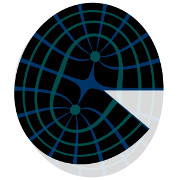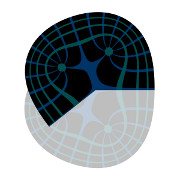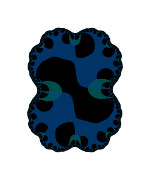Shift, double and fold, as above n = 2 n = 3 n = 6 n = 20

For each transformation, the shape is duplicated into each half of the resulting shape, so it gets twice the amount of detail or number of lumps in the shape. Some areas of the shape can shrink and rotate slightly to generate spirals, while other areas shift to make repeating fractal copies. Here is the transformation from iteration 100 to 101:n = 100 Shift, double and fold n = 101

Here are also two videos that show the process above. The second shifts by a different c at each iteration to generate a different Julia set.

To calculate Julia sets efficiently (and without quality issues from repeated image resampling) we iterate using z = z2 + c, which is equivalent to updating the coordinates to map into the previous iteration's shape. The x coordinate is the real component of z, and the y coordinate is the imaginary component. The coordinates are following the inverse of the transformations that the images went through above, and the test for |z|<2 is the same as reading from the initial image of the circle of radius 2 at the location given by the final z.

For Julia sets, c is the same complex number for all pixels, and there are many different Julia sets based on different values of c. By smoothly changing c we can transform from one Julia set to another over time, creating animated fractal shapes.

The Mandelbrot Set

For the Mandelbrot set, c instead differs for each pixel and is x + yi, where x and y are the image coordinates (as was also used for the initial z value). The Mandelbrot set can be considered a map of all Julia sets because it uses a different c at each location, as if transforming from one Julia set to another across space.

A specific Julia set can be defined by a point in the Mandelbrot set matching its constant c value, and the look of an entire Julia set is usually similar in style to the Mandelbrot set at that corresponding location. Points near the edges of the Mandelbrot set typically give the most interesting Julia sets.

Here are six Julia sets and their corresponding locations in the Mandelbrot set: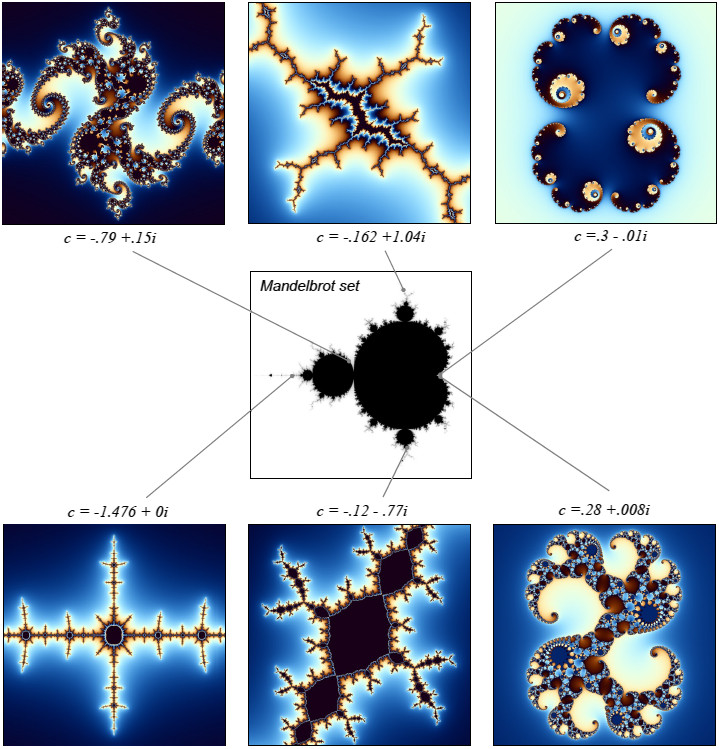Rendering Fractals

Of course we can zoom around and explore the fractal details of both Julia and Mandelbrot sets.

There are also many ways to render and colorize these fractals to give them more aesthetically interesting looks. A common method is to count the number of iterations before the magnitude of z exceeds a given escape value (usually 2) and then use that to determine the color by some color mapping technique. To smooth out the contours between colors, we can subtract a fractional amount: log2(log2|z|) from the iteration count to give a more continuous result, as shown above. It can also help to first perform an extra iteration or two after z escapes.

Another technique, referred to as the "orbit trap" method, is to check z at each iteration to see if it falls within some given shape, such as a circle, rectangle or cross, etc. If so, the color is set from the location within that shape.

In addition to these methods, there are also countless interesting ways to set a color just using the final value of z.Julia set rendered with a cross-shaped orbit trap

Note that using z = z2 + c is just one method for updating z at each iteration. If we use z3 + c, for example, we will get 3-way symmetry at each iteration instead of 2-way symmetry, which creates a whole other space of possible fractal shapes to explore.

For further information